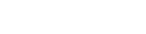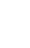# JavaScript if else statement

Conditional statements are used to do various actions in response to certain conditions.

In JavaScript, there are three types of if statements.

• if Statement
• if else statement
• if else if statement

## if statement

The if statement is executed when a condition is true.

``````
if (condition) {
//  if the condition is true, a block of code will be performed
}

``````
``````
<script>

var marks=86;

if(marks > 80 ){
}

</script>

``````

## if...else statement

The if statement is executed when a condition is true or else statement will be executed

``````
if (condition) {
//  if the condition is true
}else{
//  if the condition is false
}

``````
``````
<script>

var marks=77;

if(marks > 80 ){
}else{
}

</script>

``````

## It only analyzes the content if one or more expressions are true.

``````
if (condition 1) {
//  if the condition matches
}else if (condition 2){
//  if the condition is matches
}else{
//  if the condition is false
}

``````
``````
<script>

var marks=30;

if(marks > 80 ){
}
else if(marks > 40 ){
}
else{
document.write("Fail");
}

</script>

// Output - Fail

``````